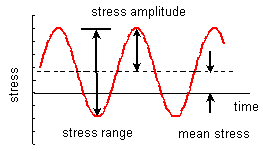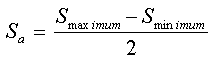Latest News

Home » Expert Opinions » Engineering Concepts » Alternating Stress – Definition and Explanation

# Alternating Stress – Definition and Explanation

What is Alternating Stress? Alternating stress is defined as the stress on a material created by forces that are changing their direction or magnitude with respect to time. This type of stress is associated with cyclic loading of a material which is a common phenomenon in machines undergoing rotational motion. The cyclic stresses can be either axial, torsional or bending in nature.

Cyclic Stresses: If we look at a typical stress diagram plotted with time we can see that the stress varies between a minimum and maximum value over a period of time. The mean stress is the average stress over the duration while the alternating stress is defined as the amount the stress varies from the mean stress.In this diagram the alternating stress is denoted as the stress amplitude. The stress range is also sometimes used to denote the alternating stress.

The formula for alternating stress is given below:Explanation of Alternating Stress with Voltage: The alternating stress is similar in principle to the alternating current where the RMS voltage is the mean value of the alternating current while the alternating stress can be equated to the value of the voltage between the RMS and the maximum voltage.

Importance in Machine Design: Alternating stresses are an important aspect of machine design since they are responsible for fatigue in a component which may cause it to fail at a stress value lower than its yield stress after a large number of cycles.Muhammad Bilal is a mechanical engineer from NUST Pakistan, he has been writing for Materialgrades.com since 2015. He has good understanding of mechanical components and engineering designs.